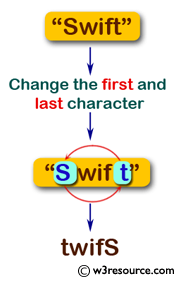﻿ Swift Basic Programming Exercise: Change the first and last character of a given string - w3resource# Swift Basic Programming Exercise: Change the first and last character of a given string

## Swift Basic Programming: Exercise-7 with Solution

Write a Swift program to change the first and last character of a given string.

Pictorial Presentation:Sample Solution:

Swift Code:

``````func first_last(str1: String) -> String {
let count = str1.characters.count

if count >= 1
{
return str1
}
var result = str1
let first_char = result.remove(at: result.startIndex)
let findLast = result.index(before: result.endIndex)
let last_char = result.remove(at: findLast)

result.append(first_char)
result.insert(last_char, at: (result.startIndex))

return result
}

print(first_last(str1: "Swift"))
print(first_last(str1: "Apple"))
print(first_last(str1: "aaaa"))
```
```

Sample Output:

```twifS
epplA
aaaa
```

Swift Programming Code Editor:

Improve this sample solution and post your code through Disqus

What is the difficulty level of this exercise?

﻿